# Oops...

This website is made for modern browsers. You are seeing this, because your browser is missing a feature or two. Please install the latest update or switch to a modern browser. See you soon.

# Four-point sail

Kangaroo Physics by Daniel Piker is a physics engine for simulation and constraint solving. It can be used within Grasshopper to find a physical equilibrium and to interactively explore different configurations of an idea. Kangaroo 2 comes preinstalled with Rhino 6 and higher and is not compatible with Kangaroo 0.099. Just like form-finding

in general, it can be used for experimental design and design optimization. Kangaroo itself based on dynamic relaxation and its components introduce different parameters to the simulation.

Using Kangaroo typically follows a similar line of action: We have to set up our experimental playground in which the magic can happen. The most important takeaway is, that we basically rebuild our model with Kangaroo components. They are called goals and each goal brings a certain energy into the system. The Kangaroo solver then tries to find the state in which the sum of all energies acting in the system is as low as possible. Every geometry or behavior that we don’t remodel will not be part of our physical simulation. This may sound obvious, but it’s the most common reason why we don’t get what we anticipated.

This tutorial needs the following plugins:

## Grasshopper

In this introductory example, we will create a four-point sail, which is an architectural membrane fixed to four points, two high and two low points. We will turn those four points into anchors, find a representation for the membrane and introduce tension to find the typical shape of a stretched fabric.

#### 1

##### Create basic geometry

To generate the four points for our sail, we will create a Center Box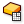Center Box (Box)
Surface  >  Primitive  >  Center Box
Create a box centered on a plane.
Inputs
Base (B)Base plane
X (X)Size of box in {x} direction.
Y (Y)Size of box in {y} direction.
Z (Z)Size of box in {z} direction.
Outputs
Box (B)Resulting box
and provide dimensions for each direction. Then, we use Box Corners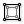Box Corners (Box Corners)
Surface  >  Analysis  >  Box Corners
Extract all 8 corners of a box.
Inputs
Box (B)Base box
Outputs
Corner A (A)Corner at {x=min, y=min, z=min}
Corner B (B)Corner at {x=max, y=min, z=min}
Corner C (C)Corner at {x=max, y=max, z=min}
Corner D (D)Corner at {x=min, y=max, z=min}
Corner E (E)Corner at {x=min, y=min, z=max}
Corner F (F)Corner at {x=max, y=min, z=max}
Corner G (G)Corner at {x=max, y=max, z=max}
Corner H (H)Corner at {x=min, y=min, z=max}
to get all vertices of this box. With Merge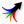Merge (Merge)
Sets  >  Tree  >  Merge
Merge a bunch of data streams
Inputs
Data 1 (D1)Data stream 1
Data 2 (D2)Data stream 2
Outputs
Result (R)Result of merge
, we can select those four vertices that will be our anchors (we have to connect them either clockwise or counterclockwise).

We will then create a mesh between our four points with Construct Mesh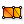Construct Mesh (ConMesh)
Mesh  >  Primitive  >  Construct Mesh
Construct a mesh from vertices, faces and optional colours.
Inputs
Vertices (V)Vertices of mesh object
Faces (F)Faces of mesh object
Colours (C)Optional vertex colours
Outputs
Mesh (M)Constructed mesh
, to set the base for our membrane. Next, we use Element* Catmull Clark SubdivisionElement* Catmull Clark Subdivision (CC)
Element*  >  Subdivision  >  Element* Catmull Clark Subdivision
Mesh based recursive subdivision defined by the Catmull Clark Algorithm
Inputs
Mesh (M)Input a polygon Mesh
Iterations (I)Input Integer specifying the Number of Subdivision Iterations
Edge Condition (E)Input integer Value list to specify edge condition type (0 = Fixed | 1 = Smooth | 2 = Corners Fixed)
Outputs
Subdivided Mesh (M)Outputs the new subdivided polygon Mesh
You could also use Weaverbird's Catmull-Clark Subdivision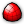Weaverbird's Catmull-Clark Subdivision (wbCatmullClark)
Wb  >  SubD  >  Weaverbird's Catmull-Clark Subdivision
Calculates the type of mesh-based recursive subdivision described by Edwin Catmull and Jim Clark, at first in their 1978 paper. The resulting mesh always consists of quad faces.

Provided by Weaverbird 0.9.0.1.
Inputs
Mesh/Curves (M)The open or closed mesh, or closed curves list, to subdivide
Level (L)The number of subdividing iterations for each face
Smooth Naked Edges (S)Defines how to treat the naked edges

0: Fixed. Naked edges will not move or be modified.
1: Smooth. The naked edge will tend toward a spline.
2: Corner Fixed. Corners (2-sided vertices) will be fixed, while other naked vertices will tend toward a spline.
Outputs
Output Mesh/Curves (O)The mesh after the subdividing process
You could also use Weaverbird's Catmull-Clark SubdivisionWeaverbird's Catmull-Clark Subdivision (wbCatmullClark)
Wb  >  SubD  >  Weaverbird's Catmull-Clark Subdivision
Calculates the type of mesh-based recursive subdivision described by Edwin Catmull and Jim Clark, at first in their 1978 paper. The resulting mesh always consists of quad faces.

Provided by Weaverbird 0.9.0.1.
Inputs
Mesh/Curves (M)The open or closed mesh, or closed curves list, to subdivide
Level (L)The number of subdividing iterations for each face
Smooth Naked Edges (S)Defines how to treat the naked edges

0: Fixed. Naked edges will not move or be modified.
1: Smooth. The naked edge will tend toward a spline.
2: Corner Fixed. Corners (2-sided vertices) will be fixed, while other naked vertices will tend toward a spline.
Outputs
Output Mesh/Curves (O)The mesh after the subdividing process
to subdivide the mesh into a higher density of mesh cells (in this case 4 iterations). The component Mesh Edges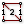Mesh Edges (MEdges)
Mesh  >  Analysis  >  Mesh Edges
Get all the edges of a mesh
Inputs
Mesh (M)Mesh for edge extraction
Outputs
Naked Edges (E1)Edges with valence 1 (a single adjacent face)
Interior Edges (E2)Edges with valence 2 (two adjacent faces)
Non-Manifold Edges (E3)Edges with valence 3 or higher
will compute the edges of all cells and sort them by their position: at output E1 we get the naked edges (those at the edges of the whole mesh) and at output E2 we get the interior edges.

#### 2

##### Reconstruct geometry as goals

With the completion of step 1, we have a geometrical description of all our objects, and we will now construct a goal for each element that needs to be included in our physical model. We start with the four points and connect them to an Anchor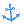Anchor (Anchor)
Kangaroo2  >  Goals-Pt  >  Anchor
Anchor
Inputs
Point (P)Point to anchor
Target (T)Location to pull the anchor to. If left empty, the initial location will be used.
Strength (Strength)Strength
Outputs
A (A)Anchor out
component; they will become fixed points.

Architectural membranes are not connected to the supports directly, but to an edges cable that is used to stretch the membrane and carry the loads to the supports. To simulate the edge cable, we attach the naked edges to a Length(Line)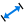Length(Line) (Length(Line))
Kangaroo2  >  Goals-Lin  >  Length(Line)
Length(Line)
Inputs
Line (Line)Line
Length (Length)Length - If none provided, starting length will be used
Strength (Strength)Strength
Outputs
Spring (S)Spring out
component and set the Strength to 1000. This component’s goal is to assign all inputted Lines the provided Length. If no length is provided, the original length is used.

For all interior edges we calculate their initial length with Length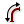Length (Len)
Curve  >  Analysis  >  Length
Measure the length of a curve.
Inputs
Curve (C)Curve to measure
Outputs
Length (L)Curve length
and then use Multiplication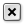Multiplication (A×B)
Maths  >  Operators  >  Multiplication
Mathematical multiplication
Inputs
A (A)First item for multiplication
B (B)Second item for multiplication
Outputs
Result (R)Result of multiplication
together with a Number Slider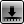Number Slider
Params  >  Input  >  Number Slider
Numeric slider for single values
to calculate the reduced lengths. We then combine everything in another Length(Line)Length(Line) (Length(Line))
Kangaroo2  >  Goals-Lin  >  Length(Line)
Length(Line)
Inputs
Line (Line)Line
Length (Length)Length - If none provided, starting length will be used
Strength (Strength)Strength
Outputs
Spring (S)Spring out
component and set the Strength to 10.

What will happen during the calculation is that the solver tries to satisfy all goals, but because they are opposed, it cannot. Thus, the stronger goals will have a greater impact. In this case, the edge cables want to keep their length and the interior edges try to shorter themselves. As the strength of the edge cables is higher, their objective is also ranked higher, and they will deform less than the interior edges. The fix points, by default, have a Strength of 10000 and therefore they deviate fewest of all.

For visualization purposes we also add a Show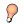Show (Show)
Kangaroo2  >  Main  >  Show
Show
Inputs
G (G)Geometry
Outputs
G (G)Connect to GoalFunctions input
component to our subdivided mesh. Technically speaking, this is not a goal, but it will pass our mesh into the solver and we get the deformed mesh as an output.

#### 3

##### Solve physical interaction

Now, we have all pieces of the puzzle and we will let Kangaroo Physics solve it by finding an equilibrium. All goals are connected to the input GoalObjects of a Solver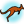Solver (Solver)
Kangaroo2  >  Main  >  Solver
The main component where Goals are combined and applied
Inputs
GoalObjects (GoalObjects)GoalObjects
Reset (Reset)Hard Reset (completely rebuild the particle list and indexing)
Threshold (Threshold)Stop when average movement is less than this (default is 1e-15)
Tolerance (Tolerance)Points closer than this distance will be combined into a single particle
On (On)If true, Kangaroo will continue to iterate until reaching the given threshold value
Outputs
I (I)Iterations
V (V)V
O (O)GoalFunction Output tree
component. We have to flatten this input to make sure all goals are in the same data branch. We also need a Button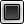Button (Button)
Params  >  Input  >  Button
Button object with two values
component for input Reset and a Boolean Toggle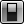Boolean Toggle (Toggle)
Params  >  Input  >  Boolean Toggle
Boolean (true/false) toggle
component for input On.

When the toggle is switched to True the solver is iterating and the current number of iterations can be found at output I. The message underneath the component states the current status of the solver, whether it is paused, running or has converged. At output O, we find our form-found objects.

## Get the results

### Version Info

As mentioned in the side note, we actually applied tension to the membrane by giving it the goal to become smaller. In reality, we would move the support nodes outwards. Now it’s your turn to model this behavior within Kangaroo.

Hint 1

### Move supports outward

We start with moving the fixed points outward. This can be done by calculating the center point of the box with VolumeVolume (Volume)
Surface  >  Analysis  >  Volume
Solve volume properties for closed breps and meshes.
Inputs
Geometry (G)Closed brep or mesh for volume computation
Outputs
Volume (V)Volume of geometry
Centroid (C)Volume centroid of geometry
and then we construct a Vector 2Pt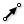Vector 2Pt (Vec2Pt)
Vector  >  Vector  >  Vector 2Pt
Create a vector between two points.
Inputs
Point A (A)Base point
Point B (B)Tip point
Unitize (U)Unitize output
Outputs
Vector (V)Vector
Length (L)Vector length
between the center and each support vertex.

We use a Move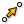Move (Move)
Transform  >  Euclidean  >  Move
Translate (move) an object along a vector.
Inputs
Geometry (G)Base geometry
Motion (T)Translation vector
Outputs
Geometry (G)Translated geometry
Transform (X)Transformation data
component, to move the points. The translation vector is set with an Amplitude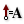Amplitude (Amp)
Vector  >  Vector  >  Amplitude
Set the amplitude (length) of a vector.
Inputs
Vector (V)Base vector
Amplitude (A)Amplitude (length) value
Outputs
Vector (V)Resulting vector
component, together with a Number SliderNumber Slider
Params  >  Input  >  Number Slider
Numeric slider for single values
, to calculate the distance for the points to travel.

Next, we have to implement this setup somehow as goals for Kangaroo.

Hint 2

### Set new supports as target

The artificial shortening of our interior edges is no longer needed and can be removed.

If we connect our displaced points as new anchor points, there would be no physical connection between them and the rest of our model. Thus, we add them as target T to our existing AnchorAnchor (Anchor)
Kangaroo2  >  Goals-Pt  >  Anchor
Anchor
Inputs
Point (P)Point to anchor
Target (T)Location to pull the anchor to. If left empty, the initial location will be used.
Strength (Strength)Strength
Outputs
A (A)Anchor out
component.

After hitting the reset button, we get the new form-found membrane.

Get the results